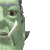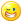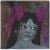## Welcome to the Second Life Forums Archive

These forums are CLOSED. Please visit the new forums HERE

# Discussion: Truly Random Hue

Abu Nasu
Code Monkey
Join date: 17 Jun 2006
Posts: 476
11-23-2006 01:14
I've spent the last few years writing my own plug-ins for Photoshop using Filter Meister. I have a rather strong background when it comes to manipulating various colour spaces. Imagine my surprise when I found out that SL and LSL are lacking when it comes to colour manipulation. The horror!

Been working on a rather bland project and needed to add some colour. The random colour scripts out there are seriously lacking for the blandness of my project. I need random colour and I mean *serious* colour. The generic random colour generators out there can generate pastels, darks, brights, and shades of grey. Those do me no good. What I need is random hue with tons of saturation. Since everything else out there seems to be lacking, seems like a good time to dip into my colour space manipulation background to learn more about LSL. After all, nothing says scripting like actually scripting.

I now have my very first script. It feels good.

It's based on the Hue <> RGB paradigm. Because of the ranges used in various places in LSL, takes advantage of bits. I don't know if it's elegant, but I think it's clever for my first script.

For a lot of uses, this is probably over-kill and probably won't be noticed. But it suits my purposes perfectly.

And it still feels good.
Cheers to learning LSL.

CODE
`// Truly Random Hue by Abu Nasu// master list for random rangeslist numbs=[7,13,19,28,49,52];// random function for floats// from the wikifloat randBetween(float min, float max){   return llFrand(max - min) + min;}// random function for integers// from the wikiinteger RandInt(integer lower, integer higher){   integer Range = higher - lower;   integer Result = llFloor(llFrand(Range + 1)) + lower;   return Result;}default{   touch_start(integer total_number)   {      // declares for this level      integer rhigh;      integer rlow;      integer ghigh;      integer glow;      integer bhigh;      integer blow;      integer rando;      integer bitMe;      float newr;      float newg;      float newb;      // get random number from master list      rando=RandInt(0,5);      bitMe=llList2Integer(numbs,rando);      // bitwise jig to get ranges for random colour function      rhigh=bitMe & 1;      rlow=(bitMe & 2 ) >> 1;      ghigh=(bitMe & 4 ) >> 2;      glow=(bitMe & 8 ) >> 3;      bhigh=(bitMe & 16 ) >> 4;      blow=(bitMe & 32 ) >> 5;      // get the random colours using ranges      newr = randBetween(rlow,rhigh);      newg = randBetween(glow,ghigh);      newb = randBetween(blow,bhigh);      // set the new colour      llSetColor(<newr,newg,newb>, ALL_SIDES);   } // end touch_start} // end default`
Nada Epoch
The LibrarianJoin date: 4 Nov 2002
Posts: 1,423
Original Thread
11-23-2006 09:55
/15/6e/150577/1.html
_____________________
i've got nothing.Lex Neva
wears dorky glasses
Join date: 27 Nov 2004
Posts: 1,361
11-23-2006 10:16
I did something like this once, but what I ended up doing to solve the problem was to port an HSL->RGB converter function to LSL and then tell it to convert an HSL value with hue uniformly random, saturation 1, and lightness 0.5. This looks a lot simpler and more efficient. Can you explain more of the technical side of how your code produces a random fully saturated color?
Abu Nasu
Code Monkey
Join date: 17 Jun 2006
Posts: 476
11-23-2006 16:50
The first thing to understand is how to define saturation. For this, used a simple difference formula.

Max=Max(R,G,B)
Min=Min(R,G,B)
Sat=Max-Min

Compare all values and get the one with the most. Compare all values and get the one with the least. Then saturation is the difference between the two. Pretty simple.

Using this definition of saturation, there is a pattern to having highly saturated colours.

Pure Red: R=1.0 G=0.0 B=0.0
Pure Orange: R=1.0 G=0.5 B=0.0
Pure Yellow: R=1.0 G=1.0 B=0.0

One value will always be 0.0, another value will always be 1.0, and the third will be somewhere inbetween. The quesion then becomes how to clamp two of the values and let the third value roam free.

Rather than nest a bunch of conditionals, why not just encode the ranges in bits?

Let's look the number 28 that can be found in numbs[]. In binary, 28 looks something like this:
00011100

Now look at it in binary like this:
00 - 01 - 11 - 00

- The two bits on the far right is the random range for the red value. In 28, red will always be 0.
- The two bits in center-right is the random range for green. In 28, green will always be 1.
- The two bits in the center-left is the random range for blue. In 28, blue will be between 0 and 1.

So, the number 28 in the list will produce a highly saturated hue between green and cyan.

It's just a matter of generating a list condusive for this method and bitwising the bits to get the ranges.

Make sense?
Joannah Cramer
Registered User
Join date: 12 Apr 2006
Posts: 1,539
11-23-2006 17:53
For the little it's worth, utilizing the full hsl to rgb model converter would go somewhat like...
CODE
`vector hsl2rgb( vector HSL ) {    // input values: hue in range 0-360. saturation and luminance in range 0-100.    float h = HSL.x;    float s = HSL.y * 0.01;    float l = HSL.z * 0.01;        if( s == 0 ) { return <l, l, l>; } // achromatic (grey)    h /= 60;    integer i = llFloor( h );          // sector 0 to 5    float f = h - i;                   // factorial part of h    float p = l * ( 1.0 - s );    float q = l * ( 1.0 - s * f );    float t = l * ( 1.0 - s * ( 1.0 - f ) );             if( i == 0 ) { return <l, t, p>; }    else if( i == 1 ) { return <q, l, p>; }    else if( i == 2 ) { return <p, l, t>; }    else if( i == 3 ) { return <p, q, l>; }    else if( i == 4 ) { return <t, p, l>; }    else if( i == 5 ) { return <l, p, q>; }    return <0.0, 0.0, 0.0>;}default {    touch_start( integer Contacts ) {	        llSetColor( hsl2rgb( <llFrand(360.0), 100.0, 100.0> ), ALL_SIDES );    }}`

... quite obviously much more plain, but hopefully the flexibility of converter can make up for it in more generic projects ^^;;
Lex Neva
wears dorky glasses
Join date: 27 Nov 2004
Posts: 1,361
11-24-2006 09:48
From: Abu Nasu

Make sense?

Yup, thanks! Very clever and concise system.
Dargon Pacer
Registered UserJoin date: 2 Jul 2005
Posts: 19
Vey nice!!!
12-14-2006 03:21
OK, I was instantly intrigued by this so I played with it a bit...
There was another script that does Gradient colors on prims and I just mixed the two together..

INSTRUCTIONS:

rez a prim, make about 5 or 6 copies nearby and link them in order..
then drop this script in it and click away!!

CODE
`// ********************************************// 'Random Gradient Hue on prims' by Dargon Pacer// (I cant take much credit for this, I just mixed two scripts together)// Original scripts:// 'Random Hue' by Abu Nasu// http://forums.secondlife.com/showthread.php?t=150577 // and // 'Gradient Colors on Prims' by MeLight Korvin// http://forums.secondlife.com/showthread.php?t=92561// pardon the ugly peice together ...figured anybody using this would // probably mod anyway// ********************************************list colCom;// master list for random rangeslist numbs=[7,13,19,28,49,52];// random function for floats// from the wikifloat randBetween(float min, float max){   return llFrand(max - min) + min;}// random function for integers// from the wikiinteger RandInt(integer lower, integer higher){   integer Range = higher - lower;   integer Result = llFloor(llFrand(Range + 1)) + lower;   return Result;}default{     state_entry()    {           }   touch_start(integer total_number)   {      // declares for this level      integer rhigh;      integer rlow;      integer ghigh;      integer glow;      integer bhigh;      integer blow;      integer rando;      integer bitMe;      float newr;      float newg;      float newb;      // get random number from master list      rando=RandInt(0,5);      bitMe=llList2Integer(numbs,rando);      // bitwise jig to get ranges for random colour function      rhigh=bitMe & 1;      rlow=(bitMe & 2 ) >> 1;      ghigh=(bitMe & 4 ) >> 2;      glow=(bitMe & 8 ) >> 3;      bhigh=(bitMe & 16 ) >> 4;      blow=(bitMe & 32 ) >> 5;      // get the random colours using ranges      newr = randBetween(rlow,rhigh);       newg = randBetween(glow,ghigh);      newb = randBetween(blow,bhigh);           // set RGB for start color values as integers and put in strings      string r1 = (string)llRound(newr);      string g1 = (string)llRound(newg);      string b1 = (string)llRound(newb);      // add a little for the gradient (second color)     // if you change the + to - in the 3 lines below, then it will      // gradient to black wich is also nice looking      string r2 = (string)llRound(newr + 1);      string g2 = (string)llRound(newg + 1);      string b2 = (string)llRound(newb + 1);            // set the new colour      string message = "grade " + r1 + " " + g1 + " " + b1 + " " + r2 + " " + g2 + " " + b2 ;           colCom = llParseString2List(message, [" "], []);        if(llList2String(colCom,0) == "grade")        {            vector startCol = <llList2Float(colCom,1), llList2Float(colCom,2), llList2Float(colCom,3)>;            vector endCol = <llList2Float(colCom,4), llList2Float(colCom,5), llList2Float(colCom,6)>;            vector grades = <(endCol.x - startCol.x)/llGetNumberOfPrims(), (endCol.y - startCol.y)/llGetNumberOfPrims(), (endCol.z - startCol.z)/llGetNumberOfPrims()>;                        integer x;            for(x = 1; x < llGetNumberOfPrims()+ 1; x++)            {                startCol += grades;                llSetLinkColor(x, startCol, ALL_SIDES);            }        }         } // end touch_start} // end default `